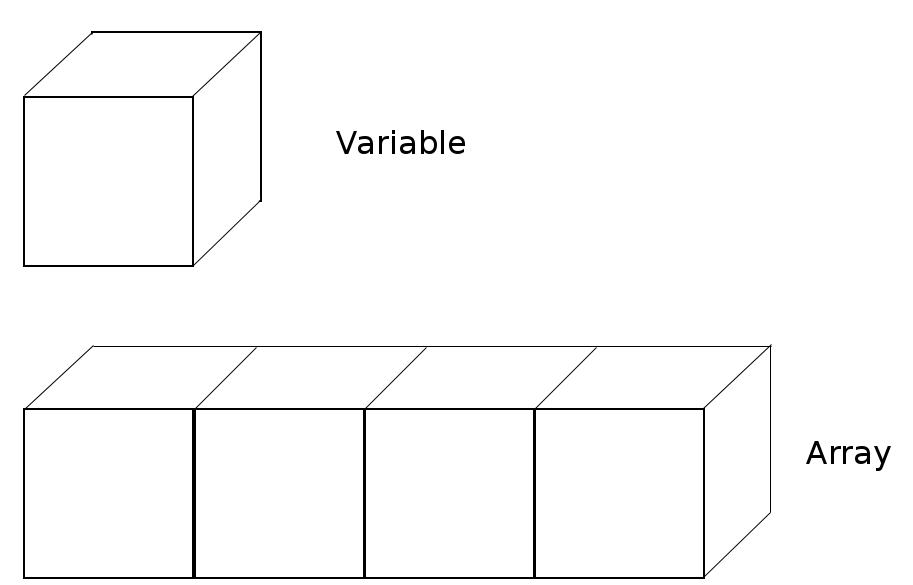# Arrays

An array is a container object that holds values of a same type.Each item in the array is called an element. Each element has a numeric index, starting with 0. To access an element, use an index and square brackets, e.g., arr. In Java, the array is an object. So, you need to create an array object.

### Examples

```int[] arr = null; //declares an array of integers.

arr = new int;
/* 4 is the size of array.
This value is stored in the length member variable of array and never change.
All elements are initialized to 0. */

arr = 1;//1st element is assigned to 1.
arr = 2;//2nd element is assigned to 2.
arr = 3;//3rd element is assigned to 3.
arr = 4;//Last element is assigned to 4.
```

Using array literals can reduce this code to a single line:

```int[] arr2 = {1, 2, 3, 4};
```
```String[] arr3 = null;//declares an array of Strings.
arr3 = new String;//The length is fixed at 2 and all elements are initialized to null.
arr3 = "Java";//1st element is assigned to "Java" object’s reference.
arr3 = "School";//2nd element is assigned to "School" object’s reference.
```

Using array literals can reduce this code to a single line:

```String[] arr4 = {"Java", "School"};
```

## To use strings in Java programs

How to express the strings in Java is to create a String object from the String class. If you need the string "Hello", code it like this:

```char[] arrayOfHello = {'H','e','l','l','o'};
String greetings = new String(arrayOfHello);
```

Java provides String literals.

```String hello = "Hello"; //Here, "Hello" is a String Literal.
```

### How to use String[] parameter in main method

```public class ArrayTest {
public static void main(String[] args) {
System.out.print(args + "/");
System.out.print(args + "/");
System.out.println(args);
}
}
```
C:\ Command Prompt
```C:\javaApp>javac ArrayTest.java

C:\javaApp>java ArrayTest 2018 12 31
2018/12/31
```